#Quality Digest

Featured Product
This Week in Quality Digest Live
Quality Insider Features
NIST
Don’t underestimate the power of telling your story
Oak Ridge National Laboratory
Hafnium oxide key to novel applications
William A. Levinson
Deciding whether you need CAPA or a bigger boat
Matthew T. Hughes
Two engineers explain how heat waves threaten everything from cars to computers
Baxi Chong
Can quantity equate to quality?
Quality Insider News
To be unveiled during PACK EXPO Las Vegas at the Hiperbaric booth, No. N-10857
Educating the next generation of machinists with state-of-the-industry equipment
In a first, researchers have observed how lithium ions flow through a battery interface
Air-extend/spring-retract moves probe out of the way
Precision cutting tools maker gains visibility and process management across product life cycles
Expanded offering includes Smartscope E-series for 3-axis video measurement
Accelerates CAM programming time 80% to make U.S. manufacturers more productive
Pioneers new shape-memory alloys
A Heart for Science initiative brings STEM to young peopleBio

Quality Insider

## The Analysis of Experimental Data

### Minimizing the complexity improves communication

Published: Monday, January 6, 2014 - 13:26

You have spent good money obtaining your experimental results, and now the time has come to communicate those results to those who need to take action. This column will describe how to cut through the complexities of your analysis and communicate the results quickly and easily.

You have 30 seconds to make your point before your boss’s eyes glaze over, and the easiest way to beat this 30-second rule is to use a graph. In thinking about how to beat the 30-second rule Ellis Ott decided to combine the graphic power of the average and range chart with the sensitivity of the analysis of variance (ANOVA). The result was the analysis of means (ANOM). The original version in 1967 used approximate critical values to compute the ANOM decision limits. Over the years several investigators have worked on sharpening up and extending these decision limits. The tables given here are excerpted from the results of the latest such revision carried out during the past two years. However, before we get to the ANOM, we shall begin with the traditional ANOVA.

### The analysis of variance (ANOVA)

We begin with the data for a two-factor experiment. Two concentrations and five processing temperatures are examined for their effects upon the reflectivity of a certain coating. Three samples are coated and processed using each of the ten combinations of concentration and temperature. The data are shown in figure 1. When these data are placed in an appropriate software package you will get the ANOVA table of figure 2 as your output.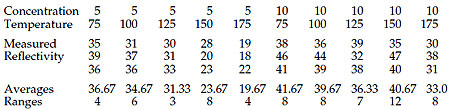Figure 1: The reflectivity data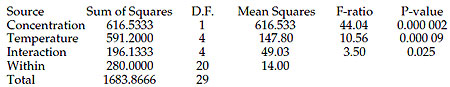Figure 2: The ANOVA table for the reflectivity data

When using this ANOVA table to present your results you might tell your boss that both the concentration and the temperature have an effect upon the reflectivity, and that there is also an interaction effect as well. Although this would be true, it would be incomplete. Three important questions that the ANOVA table does not answer are: How does the concentration level affect the reflectivity?, How does the temperature affect the reflectivity? And, how does the interaction effect change the reflectivity? The ANOVA table tells us which effects are likely to be real, but it does not show the actual effects. Other tools or techniques are needed to answer these questions. However, a simple ANOM chart will detect the effects and show the relationships at the same time.

### Analysis of means (ANOM) and analysis of ranges (ANOR)

The analysis of means is much simpler than the ANOVA. We compute the detection limits just like we compute limits for an average and range chart. The grand average for the data in figure 1 is 33.733 and the average range is 6.80. With an overall alpha level of 10 percent the ANOM scale factor for k = 10 subgroups of size n = 3 from Table A (scroll to end of article for all tables) is 0.893, so the ANOM detection limits are:

Grand Average ± ANOM.10 (Average Range) = 33.733 ± 0.893 (6.8) = 27.66 to 39.81

The analysis of ranges (ANOR) proceeds in a similar manner. With an alpha level of 5 percent, and with k = 10 and n = 3, the ANOR scaling factor is found in Table B to be 2.519. Thus, the ANOR upper detection limit is:

ANOR.05 (Average Range) = 2.519 (6.8) = 17.1

The resulting ANOM plot is shown in figure 3.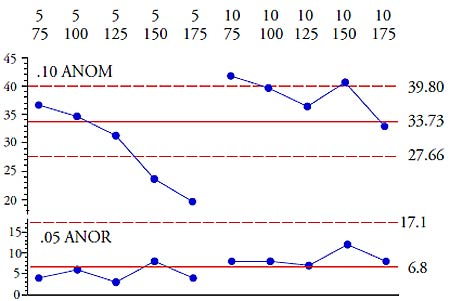Figure 3: ANOM chart for the reflectivity data

The ANOM chart in figure 3 looks like an average and range chart, and it’s as easy to construct as an average and range chart, yet it provides an analysis for your experimental data that uses your preferred alpha level. In this case the ANOM uses an exploratory alpha level of 10 percent, while the ANOR uses a traditional alpha level of 5 percent. (With the usual three-sigma limits the ordinary average chart for these data would have an alpha-level of 4.2%, while the range chart would have an alpha level of 4%.) Since the graphs look the same, I am always careful to label my ANOM and ANOR charts as such and give the alpha level used.

In addition to being easy to construct, the ANOM chart is also easy to interpret. Four treatment averages are identified as being detectably different from the grand average, and there is no evidence of any differences in the within-treatment variation on the ANOR chart. By leaving a gap between points five and six we make it easy to see that the reflectivity goes up with concentration; the reflectivity goes down with temperature; and at the high concentration and higher temperatures there is an unexpected increase in reflectivity. This interaction effect can be exploited or avoided as necessary only after it has been visualized.

But how can we be sure that there are main effects for both concentration and temperature? These can be validated by using a follow-up analysis of main effects (ANOME).

### Analysis of main effects (ANOME)

When a multifactor study is carried out using a fully-crossed design it will be easy to create main-effect charts for each of the factors in the study. In the example considered here, the concentration factor has two levels: 5 grams per liter and 10 grams per liter. The ANOME for concentration will compare the average response for each of these two levels with the grand average. So the first step is to compute the average response for each level of concentration.

The average for the five treatments that had concentrations of 5 grams per liter is 29.20.

The average for the five treatments that had concentrations of 10 grams per liter is 38.27.

The ANOME scaling factor for comparing m = 2 averages with an alpha-level of 10 percent when the original study had k = 10 and n = 3 is found in Table C to be 0.184. The ANOME decision limits are:

Grand Average ± ANOME.10 (Average Range) = 33.73 ± 0.184 (6.8) = 32.48 to 34.98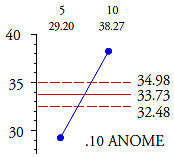Figure 4: ANOME chart for concentration main effect

Clearly, there is a real effect here and the reflectivity goes up nine units on the average as the concentration goes from 5 to 10.

The temperature factor has five levels. The ANOME for temperature will compare the average response for each of these five levels with the grand average. So we compute the average response for each level of temperature.

The average for the two treatments that had temperatures of 75 degrees is 39.17.

The average for the two treatments that had temperatures of 100 degrees is 37.17.

The average for the two treatments that had temperatures of 125 degrees is 33.83.

The average for the two treatments that had temperatures of 150 degrees is 32.17.

The average for the two treatments that had temperatures of 175 degrees is 26.33.

The ANOME scaling factor for comparing m = 5 averages with an alpha-level of 10 percent when the original study had k = 10 and n = 3 is found in Table C to be 0.526. The ANOME decision limits are:

Grand Average ± ANOME.10 (Average Range) = 33.73 ± 0.526 (6.8) = 30.16 to 37.31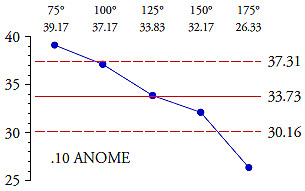Figure 5: ANOME chart for temperature main effect

Clearly, there is a real effect here and the reflectivity goes down about 13 units on the average as temperature goes from 75 to 175.

Figures 4 and 5 confirm the impression given by figure 3: Both concentration and temperature have an effect upon reflectivity. But how can we be sure about the interaction effect? The ANOVA is the easiest place to determine if a particular interaction effect is likely to be real, but the ANOM plot is the easiest place to interpret what the interaction effect means in terms of the experimental results. All interaction effects must show up on the ANOM plot as non-parallelness between corresponding line segments. Looking at the two curves from the ANOM plot in figure 6, the two segments going from 75 degrees to 100 degrees are perfectly parallel, as are the two segments going from 100 to 125. However, the two segments going from 125 to 150 are almost perpendicular to each other. Finally the two segments going from 150 to 175 are reasonably parallel.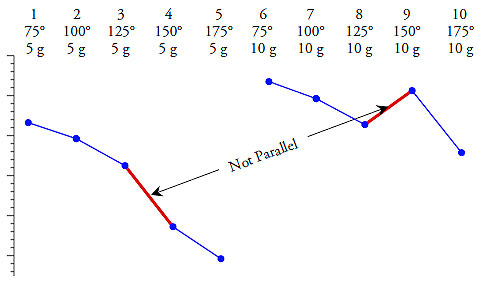Figure 6: Interactions must show up as non-parallel line segments

Thus, the interaction effect detected in the ANOVA table consists of elevated reflectivities for the higher temperatures and high concentration treatment combinations (subgroups 9 and 10).

### Summary

ANOM, ANOR, and ANOME allow you to analyze your experimental data with a minimum of effort. They provide you with a picture to use in presenting your results. And they allow you to focus the discussion on the interesting aspects of your data rather than getting bogged down in, or even misled, by a discussion of the complexities of the analysis technique. This approach will minimize the time and effort required in order to begin to use your experimental data effectively. If you haven’t started using ANOM, you simply do not know what you have been missing.

(This example comes from Analyzing Experimental Data [SPC Press, 2013]. The following tables are excerpted from larger tables given there. Both the example and the tables are used with permission.)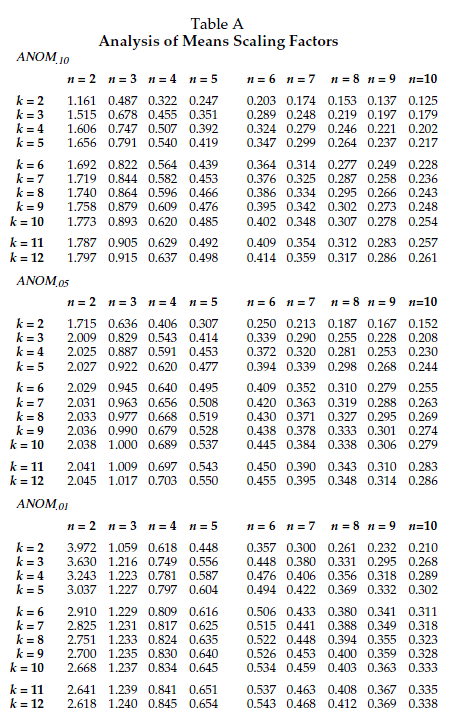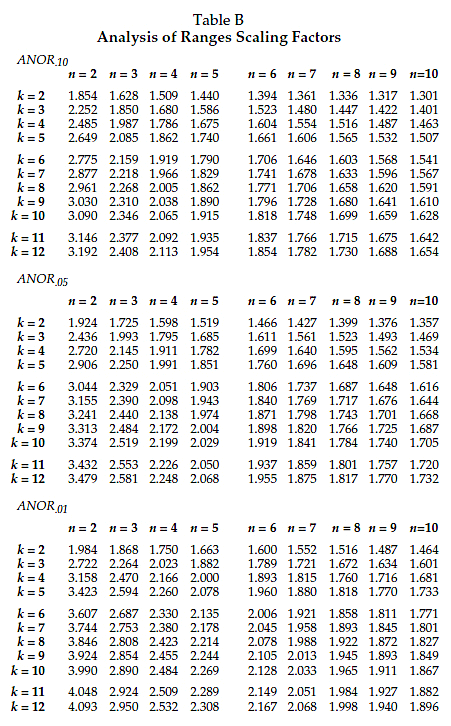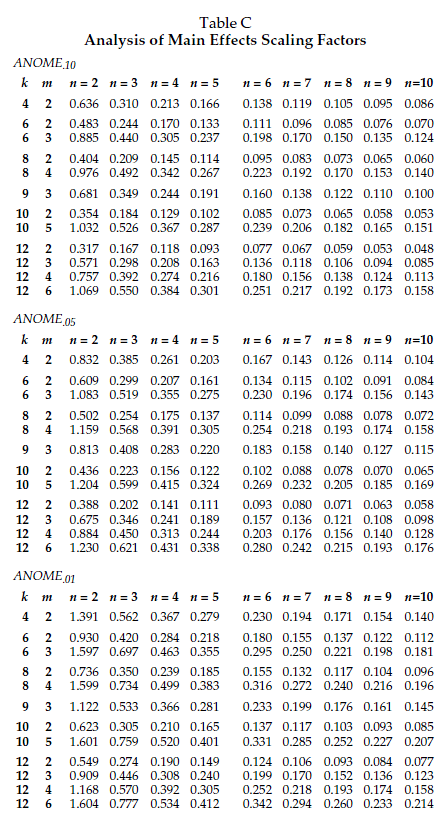Donald J. Wheeler is a Quality Digest content partner.

### Discuss### Donald J. Wheeler

Dr. Wheeler is a fellow of both the American Statistical Association and the American Society for Quality who has taught more than 1,000 seminars in 17 countries on six continents. He welcomes your questions; you can contact him at djwheeler@spcpress.com.

### The Analysis of Experimental Data

Hi Dr Wheeler,

Interesting article. There is a fourth question that could/should be answered from this data. That is the process optimisation question. For example, if we require Y as an output value for reflectivity, what would be the optimal X input values for both concentration and temperature?  This is easily answered by statistical software, where the mathematical relationship can be determined.

### Question on Range Chart Scaling Factor

Hello Dr. Wheeler,

Your post provided a slightly different approach to the example in "Understanding Industrial Experimentation". It's a cleaner way to determine the decision limits using Table A & the average R. Also, your point made in Fig 6. was much clearer. So, thank you for that.

I did have a question with respect to the range chart and the way the range limit is determined. I used the control chart factor D4 to scale the average R to get the upper limit. For n = 3, D4 = 2.574. However, in your post you use 2.519. Can you help me understand the difference?

One other question: Is it possible for a difference to show up in the ANOVA and not in the ANOM? Is one approach more sensitive or less robust than the other?

To all others:

Dr. Wheeler's book on industrial experimentation is outstanding. I cannot recommend it enough. It helped me learn experimentation methods and put them to use quickly. It's obvious he spent a lot of time trying to view the material from the student's perspective. You should use this book before diving into the classics like "Statistics for Experimenters".

### Too complicated

If it were me having the test results presented to me, I would want to see a simple line graph with reflectivity on the y axis, temperature and the x axis, and a line for each concentration.  In one view of the chart I could understand.  All that needs to be communicated would be there.

### Genuinely Curious

Hi Dave,

Maybe I'm missing something, but isn't Fig. 3 just the chart you're describing, but without decision limits?

Without decisions limits, how would you determine if the differences were real?

Without the ANOME charts, how would you determine the size of the main effects?

My questions are out of genuine curiosity. If you have a better faster easier way to determine these things, I would love to learn them and put them to use.